## Parametric Equation Oscillators

Hans Mikelson

hljmm@werewolf.net

Introduction

Parametric equations used in generating polar graphs are also useful in generating interesting sounds. These curves make interesting sound sources for synthesis because they typically have a variety of parameters which can be modulated. Since there are two or more outputs they naturally generate stereo signals.

Cycloids

Cycloid curves, sometimes called trochoids, are created by a circle rotating inside or outside of another circle. Cycloid curves were made popular by Hasbro's game Spirograph which consisted of various disks with gear teeth on the edges. A pen was used to trace the curve of the cycloid through holes in the disks. The parametric equations defining these curves are:Where a is the radius of the stationary circle and b is the radius of the rotating circle. If s is +1 it represents a circle rotating outside another circle and if s is -1 it represents a circle rotating inside of the other circle. The parameter h represents the point on the rotating disk from which the curve is traced. This would correspond to the hole in a Spirograph game although in this case the "hole" can extend beyond the radius of the rotating circle.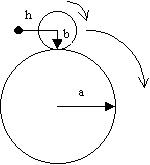```Figure 1
Diagram of a cycloid```

Some of the curves possible with this type of system follow: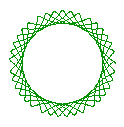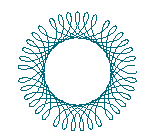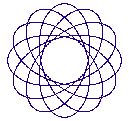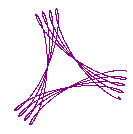```Figure 2
Four different cycloid curves```

This system can be implemented in Csound as:

``` ; Sine and Cosine
acos1    oscil  ia+ib*isgn, afqc, 1, .25
acos2    oscil  ib*ihole, (ia-ib)/ib*afqc, 1, .25
ax       =      acos1 + acos2

asin1    oscil  ia+ib*isgn, afqc, 1
asin2    oscil  ib*ihole, (ia-ib)/ib*afqc, 1
ay       =      asin1 - asin2```

The x and y values are then output as the left and right channels to create the tone. This sound works well with pitch effects like portamento, vibrato, pitch envelopes and pitch bending.

Butterfly Curves

Butterfly cureves look a little like a butterfly in shape. Butterfly curves are generated by the following equations: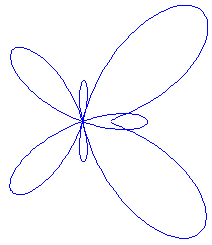```Figure 1.
Butterfly Curve a=2, b=4, c=5, d=12 and a=2.1, b=6, c=7, d=30```

This can be implemented in Csound as:

```; Cosines
acos1  oscil 1,  ifqc,    1, .25
acos2  oscil ia, kb*ifqc, 1, .25
acos3  oscil 1, ifqc/kd,   1, .25

; Sines
asin2  pow asin1, ic
asin3  oscil 1, ifqc/kd,   1

arho   =     exp(ie*acos1)-acos2+asin2

ax     =     arho*acos3
ay     =     arho*asin3```

Using a very low frequency for the cos3 and sin3 terms above results in a slowly evolving stereo image.

Spherical Lissajous Curves

The next system considered is the spherical lissajous curve. The image on the cover of this magazine is a spherical lissajous curve. The following equations define this curve: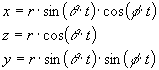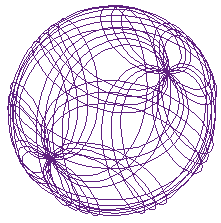```Figure 3.
Spherical lissajous curves```

The Csound code to implement this is:

```; Cosines
acosu   oscil   1, iu*ifqc,   1, .25
acosv   oscil   1, iv*ifqc,   1, .25

; Sines
asinu   oscil   1, iu*ifqc,   1
asinv   oscil   1, iv*ifqc,   1

; Compute X and Y